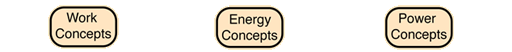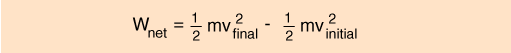# Power

### is the rate of doing work or the rate of using energy, which are numerically the same. If you do 100 joules of work in one second (using 100 joules of energy), the power is 100 watts.Index

Work concepts

 HyperPhysics***** Mechanics R Nave
Go Back

# Work-Energy Principle# The change in the kinetic energy of an object is equal to the net work done on the object.

This fact is referred to as the Work-Energy Principle and is often a very useful tool in mechanics problem solving. It is derivable from conservation of energy and the application of the relationships for work and energy, so it is not independent of the conservation laws. It is in fact a specific application of conservation of energy. However, there are so many mechanical problems which are solved efficiently by applying this principle that it merits separate attention as a working principle.

For a straight-line collision, the net work done is equal to the average force of impact times the distance traveled during the impact.

### Average impact force x distance traveled = change in kinetic energy

If a moving object is stopped by a collision, extending the stopping distance will reduce the average impact force.

## Work-energy principle for angular quantities

The rate of doing work is equal to the rate of using energy since the force transfers one unit of energy when it does one unit of work. A horsepower is equal to 550 ft lb/s, and a kilowatt is 1000 watts.
Index

Example

 HyperPhysics***** Mechanics R Nave
Go Back